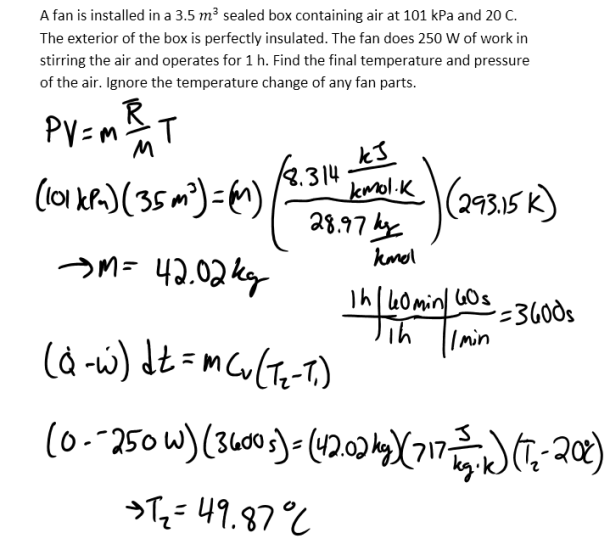heat and mass transfer problems engineering equations heat and mass transfer school homework engineering heat and mass transfer formulas heat and mass transfer solutions to heat and mass transfer problems full solution engineering problem solution heat and mass transfer math problems engineering equations heat and mass transfer school homework engineering solutions to heat and mass transfer formulas heat problems mass problem solutions to transfer problems full solution heat and mass transfer
heat and mass transfer problems engineering equations heat and mass transfer school homework engineering heat and mass transfer formulas heat and mass transfer solutions to heat and mass transfer problems full solution engineering problem solution heat and mass transfer math problems engineering equations heat and mass transfer school homework engineering solutions to heat and mass transfer formulas heat problems mass problem solutions to transfer problems full solution heat and mass transfer
Highalphabet Home Page heat and mass transfer problem solutions Heat and Mass Transfer Page
A fan is installed in a 3.5 m^3 sealed box containing air at 101 kPa and 20 C. The exterior of the box is perfectly insulated. The fan does 250 W of work in stirring the air and operates for 1 h. Find the final temperature and pressure of the air. Ignore the temperature change of any fan parts.A fan is installed in a 3.5 m^3 sealed box containing air at 101 kPa and 20 C. The exterior of the box is perfectly insulated. The fan does 250 W of work in stirring the air and operates for 1 h. Find the final temperature and pressure of the air. Ignore the temperature change of any fan parts.